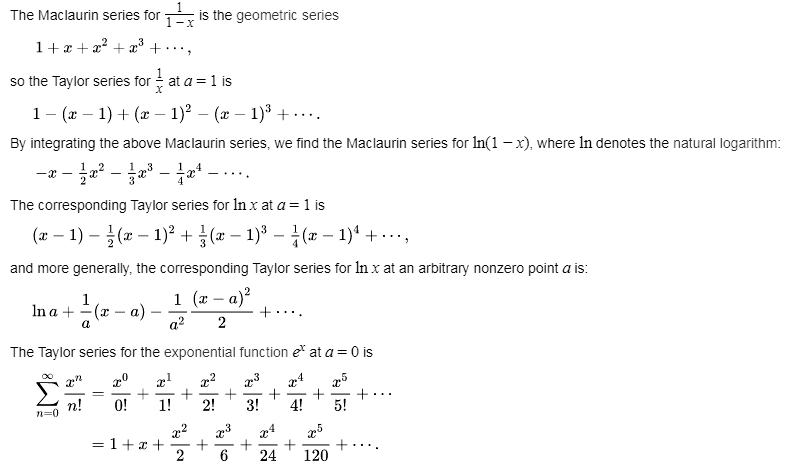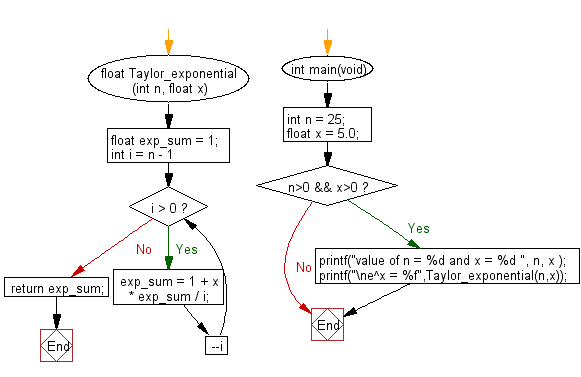﻿ C Program: Sum first n terms of Taylor series for e^x

# C Exercises: Calculate e raise to the power x using sum of first n terms of Taylor Series

## C Programming Mathematics: Exercise-24 with Solution

Write a C program to calculate e raised to the power of x using the sum of the first n terms of the Taylor Series.

From Wikipedia,
In mathematics, a Taylor series is a representation of a function as an infinite sum of terms that are calculated from the values of the function's derivatives at a single point.
Example:
The Taylor series for any polynomial is the polynomial itself.The above expansion holds because the derivative of ex with respect to x is also ex, and e0 equals 1.
This leaves the terms (x − 0)n in the numerator and n! in the denominator for each term in the infinite sum.

Example:
Input: n = 25
float x = 5.0
Output: e^x = 148.413162

Sample Solution:

C Code:

``````#include <stdio.h>
#include <stdlib.h>

float Taylor_exponential(int n, float x)
{
float exp_sum = 1;
for (int i = n - 1; i > 0; --i )
exp_sum = 1 + x * exp_sum / i;
return exp_sum;
}
int main(void)
{
int n = 25;
float x = 5.0;

if (n>0 && x>0)
{
printf("value of n = %d and x = %d ", n, x );
printf("\ne^x = %f",Taylor_exponential(n,x));
}
}
```
```

Sample Output:

```value of n = 25 and x = 1968710504
e^x = 148.413162
```

Flowchart:C Programming Code Editor:

Improve this sample solution and post your code through Disqus.

What is the difficulty level of this exercise?

Test your Programming skills with w3resource's quiz.

﻿